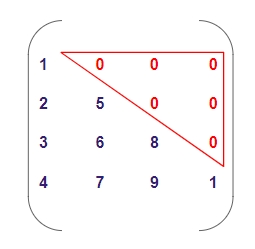# Program to check if matrix is lower triangular in C++

Given a square matrix M[r][c] where ‘r’ is some number of rows and ‘c’ are columns such that r = c, we have to check that ‘M’ is lower triangular matrix or not.

## Lower Triangular Matrix −

Lower triangular matrix is a matrix in which the elements below the main diagonal(including the main diagonal) are not zero and above elements are zero only.

Like in the given Example below −In above figure the red highlighted elements are upper elements from the main diagonal which are zero and rest elements are non-zero.

## Example

Input: m = { {1, 0, 0},
{2, 3, 0},
{4, 5, 6}}
Output: yes
Input: m == { {3, 0, 1},
{6, 2, 0},
{7, 5, 3} }
Output: no

## Algorithm

Start
Step 1 -> define macro as #define size 4
Step 2 -> declare function to check matrix is lower triangular matrix
bool check(int arr[size][size])
Loop For int i = 0 and i < size and i++
Loop For int j = i + 1 and j < size and j++
If (arr[i][j] != 0)
return false
End
End
End
return true
step 3 -> In main()
Declare array int arr[size][size] = { { 1, 0, 0, 0 },
{ 2, 3, 0, 0 },
{ 4, 5, 6, 0 },
{ 7, 8, 9, 10 } }
If (check(arr))
Print its a lower triangular matrix
Else
Print its not a lower triangular matrix
Stop

## Example

#include <bits/stdc++.h>
#define size 4
using namespace std;
// check matrix is lower triangular matrix
bool check(int arr[size][size]){
for (int i = 0; i < size; i++)
for (int j = i + 1; j < size; j++)
if (arr[i][j] != 0)
return false;
return true;
}
int main(){
int arr[size][size] = { { 1, 0, 0, 0 },
{ 2, 3, 0, 0 },
{ 4, 5, 6, 0 },
{ 7, 8, 9, 10 } };
if (check(arr))
cout << "its a lower triangular matrix";
else
cout << "its not a lower triangular matrix";
return 0;
}

## Output

its a lower triangular matrix